## Introduction to Discrete Groups via Multiplication Tables

Group theory is a proven tool for identifying and using symmetries in mathematics and physics. Introductory materials for teaching groups and group theory, seem to me, to be in too much of a hurry to get to modern topics. Specifically, I feel we need to include much more material using multiplication tables and Cayley diagrams in the introductory material for groups. By developing and presenting the comprehensive list of groups through order 10 or so, we motivate and justify concepts such as generators, and irreducible representations.

### Recommended Free Software

There are two pieces of software that I highly recommend for the introductory group class. These are Group Explorer, by Nathan Carter, and GAP,  a world-wide project, downloadable from www.gap-system.org.

Nathan Carter at Bentley University in Waltham, Massachusetts has written and published Group Explorer software for Windows, Macintosh, and Linux. The download URL is

http://sourceforge.net/projects/groupexplorer/

Group Explorer provides a listing of the smallest groups, their names, their multiplication tables, Cayley diagrams, Cayley graphs and generators in an attractive, interactive format.

GAP, on the other hand, is the authoritative reference for small groups (rank less than 1024), but makes no pretense about being casual user friendly. (Of course, after the software has you trained, you will find it very user friendly!)

### Group Properties

Two groups, the symmetric group and the cyclic group, figured prominently in the early study of groups.

#### The Symmetric Group

The study of the permutations arose from the study of the roots of polynomial equations. The coefficients of the polynomial are symmetric functions of the roots, taken one at time, then two at a time, and so on. These coefficients are invariant under permutations of the roots.
As an example, the set of coefficients for the cubic equation are

x3 + Ax2 + Bx + C = (x - r1)*(x - r2)*(x - r3) = 0

A = -(r1 + r2 + r3)

B = (r1*r2 + r2*r3 + r3*r1)

C = -r1*r2*r3

Swapping r1, r2 and r3 among themselves has absolutely no effect on the values of A, B and C. Likewise, any function made from the A, B, and C will inherit invariance under permutation of the roots.

Permutations feature:
• an identity element, which is no permutation at all,
• an inverse for each element, which undoes the previous permutation,
• closure, where the result of two cascaded permutations is another permutation, and
• associativity, where the result of three cascaded operations is insensitive to the ordering of parenthesis creating binary operations. (A.B).C = A.(B.C)

As we generalized from permutations to groups, the four properties above carried forth.

The common naming convention uses a letter S, to indicate Symmetric, followed by a number, which gives the set size of the object being permuted. The number of permutations of a set of n elements is n!, so each permutation group has a size, or order, of n! permutations. S2 has two members, S3 has six members, S4 has 24 members, S5 has 120 members, and so on. Other notation I've seen includes Sym for symmetric, as well as P for permutation.

#### The Cyclic Group

Whereas the symmetric group is limited to orders of n!, the cyclic group can be found at any size. Historically, the cyclic group of size N was the set of the N complex roots of 1. When N is a prime number, the cyclic group is the only group of that size available. Two common notations for the cyclic group are used. Gap uses the CN notations, such as C3 for the cyclic group of order 3, while Group Explorer uses ZN, such as Z3 for the same group.

### The Smallest Groups

We now are going to walk through the smallest groups, introducing topics along the way.

#### S2,  C2 and Z2 - Cyclic Group and Symmetric Group With Two Elements

This smallest of sets has two members. On the left is the GAP multiplication table, where the numbers 1 and 2 can be used as indices into a table of letters. To the right, is a generic multiplication
table, using A and B as representations of group members.

`GAP Multiplication Table       S2/C2/Z2 - ASCII Multiplication Table                            (Prefactor left column, Postfactor top row)                                   _|_A_B     _|_I_A     __|_+1_-1_[ [ 1, 2 ],                        A| A B     I| I A     +1| +1 -1  [ 2, 1 ] ]                       B| B A     A| A I     -1| -1 +1`
You notice that the first row matches the top heading, and the left column matches the left heading. This identifies A as the identity element. Traditionally, the letters E or I are used for this element. Traditionally, we start the standard form of a group multiplication table with the identity top and left.

Next, we notice the same table, this time using I for the unit, and A for the other element (which used to be B). Finally, we have a faithful representation of this group, using multiplication for the group action, and +1 and -1 as group elements. As this is a cyclical group of order 2, we see we are dealing with square roots of 1. Alternatively, we can call out A = e(i2π/2) ,  and I =1 = A*A = A2.

Group Explorer show a colored multiplication table without headings, but includes the generators in the table cells. The definition to the right of Z2 says we have one generator, a, with the relationship
a2 = 1.

Z2 : <a:a2=1>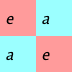Returning to the ASCII multiplication table, we see that this particular table is organized with a unit diagonal from top right to lower left. This implies that the left column is the inverse of the top row. We can obtain a faithful matrix representation of the group elements when we have such an arrangement as shown below. We replace all instances of the desired group member with a 1, and zero out all other cells. Using standard matrix multiplication, we verify A*A = I, I*A = A*I = I.

`_|_I_AI| I A      I =>  1 0       A => 0 1A| A I            0 1            1 0`
As we look at the multiplication table, we see it is symmetric around the descending diagonal. Consequently, this group is commutative as well as associative. To help lower comprehension, rather than call out a group as commutative, which clearly identifies the property of interest, we instead call commutative groups Abelian, after Niels Henrik Abel (5 August 1802 – 6 April 1829).

#### C3 - Cyclic Group With Three Elements

The only group for (prime) order 3 is the cyclic group corresponding to cube roots of 1. The GAP command to show the multiplication table for C3 is shown on the top line.

`MultiplicationTable(SmallGroup(3,1));                                _|_I_A_B[ [ 1, 2, 3 ],                I| I A B  [ 2, 3, 1 ],                A| A B I  [ 3, 1, 2 ] ]               B| B I A`
The definition for this group uses one generator, a, with <a:a3=1>. My elements in the right hand truth table are I =  a3, A = a, and B = A*A = A2 = a2.

From Group Explorer, we have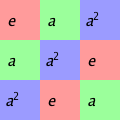Using the knowledge that a3=1, we can use complex numbers (Euler's or De Moivre's Theorem) to write

A = e(i2π/3) = cos(120°) + i sin(120°) = 0.5 + i 0.866
B = A2 = e(i4π/3) = cos(240°) + i sin(240°) = 0.5 - i 0.866
I = A*B = A3 =  e(i6π/3) = e(i2π) = 1.0

How about a matrix representation for the group elements? We will need to re-arrange our multiplication table to have a descending unit diagonal, (from which we can easily read inverse elements), and then we can read off the matrix representation as done for C2/Z2/S2 above.

`_|_I_A_B  Our original table. Notice A^2 = B. B^2 = A.I| I A B                 Also notice A^3 = 1. B^3 = 1.A| A B IB| B I A_|_I_A_B   We see A and B are inverse to each other.I| I A B   1/A = B, and 1/B = AB| B I AA| A B I    1 0 0        0 1 0        0 0 1I = 0 1 0    A = 0 0 1    B = 1 0 0    0 0 1        1 0 0        0 1 0`

#### C4 - Cyclic Group With Four Elements

We have two groups of order 4, being the cyclic group C4 and the Klein four-group, or Vierergruppe. For the C4 group, I've included the GAP prompt, as well a command for the structural description of this group. The ASCII table is just to the right of the GAP output.

```gap> StructureDescription(SmallGroup(4,1)); "C4"gap> MultiplicationTable(SmallGroup(4,1));                            _|_I_A_B_C[ [ 1, 2, 3, 4 ],          I| I A B C  [ 2, 3, 4, 1 ],          A| A B C I  [ 3, 4, 1, 2 ],          B| B C I A  [ 4, 1, 2, 3 ] ]         C| C I A B_|_I_A_B_C    A = 1^(1/4) = i   I| I A B C    B = A^2 = 1^(1/2) = i^2 = -1
A| A B C I    C = AB = BA = 1^(3/4) = -i
B| B C I AC| C I A B_|_I_A_B_C    We see 1/A = C, !/B = B and 1/C = AI| I A B CC| C I A BB| B C I AA| A B C IMatrix Representation    1 0 0 0       0 1 0 0       0 0 1 0       0 0 0 1I = 0 1 0 0   A = 0 0 1 0   B = 0 0 0 1   C = 1 0 0 0    0 0 1 0       0 0 0 1       1 0 0 0       0 1 0 0    0 0 0 1       1 0 0 0       0 1 0 0       0 0 1 0```
From Group Explorer, we have the generator relationship <a:a4=1>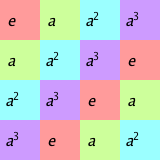#### V4 - ViererGruppe or Klein Group - C2 x C2

The Klein 4-group also is of order four, but can be thought of as two copies of C2 acting independently of each other.

`gap> StructureDescription(SmallGroup(4,2));"C2 x C2"gap> MultiplicationTable(SmallGroup(4,2));                         _|_I_A_B_C[ [ 1, 2, 3, 4 ],       I| I A B C  [ 2, 1, 4, 3 ],       A| A I C B  [ 3, 4, 1, 2 ],       B| B C I A  [ 4, 3, 2, 1 ] ]      C| C B A I_|_I_A_B_C   We see A^2 = B^2 = C^2 = 1I| I A B C   AB = BA = CA| A I C B   AC = CA = BB| B C I A   BC = CB = AC| C B A IWe are already in the unit diagonal format, so    1 0 0 0       0 1 0 0       0 0 1 0       0 0 0 1I = 0 1 0 0   A = 1 0 0 0   B = 0 0 0 1   C = 0 0 1 0    0 0 1 0       0 0 0 1       1 0 0 0       0 1 0 0    0 0 0 1       0 0 1 0       0 1 0 0       1 0 0 0`
From Group Explorer, we have the generators <a,b:a2=b2=1,ab=ba>. They don't list c=ba, as this would imply the presense of a third generator for this family. Also, notice the relationship between products ab=ba. This relationship will be different, for example, for the dihedral family of groups.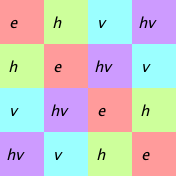As we look at this table, we see the choice of letters is h = horizontal flip and v = vertical flip.

This is our first group to include subgroups. Look at the re-organized tables below. Notice how the upper left hand corner can be a self-contained group? These are the three subgroups of V4, being (I,A) , (I,B) and (I,C).

`   Subgroups of V4 - Klein four-group_|_I_A__B_C    _|_I_B__C_A    _|_I_C__A_BI| I A  B C    I| I B  C A    I| I C  A BA| A I  C B    B| B I  A C    C| C I  B AB| B C  I A    C| C A  I B    A| A B  I CC| C B  A I    A| A C  B I    B| B A  C I`

#### C5 - Cyclic Group With Five Elements

Being that five is a prime number, we only have one cyclic group.

`MultiplicationTable(SmallGroup(5,1));                           _|_I_A_B_C_D[ [ 1, 2, 3, 4, 5 ],       I| I A B C D  [ 2, 3, 4, 5, 1 ],       A| A B C D I  [ 3, 4, 5, 1, 2 ],       B| B C D I A  [ 4, 5, 1, 2, 3 ],       C| C D I A B  [ 5, 1, 2, 3, 4 ] ]      D| D I A B C`
No subgroups are present. We have just one generator.  <a:a5=1>

From Group Explorer, the colored multiplication table is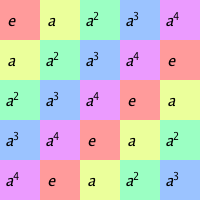The inverse map for C5, and the matrix representation are shown below.
`_|_I_A_B_C_DI| I A B C D   AD = ID| D I A B C   BC = IC| C D I A BB| B C D I AA| A B C D I     1 0 0 0 0       0 1 0 0 0       0 0 1 0 0       0 0 0 1 0       0 0 0 0 1     0 1 0 0 0       0 0 1 0 0       0 0 0 1 0       0 0 0 0 1       1 0 0 0 0I =  0 0 1 0 0   A = 0 0 0 1 0   B = 0 0 0 0 1   C = 1 0 0 0 0   D = 0 1 0 0 0     0 0 0 1 0       0 0 0 0 1       1 0 0 0 0       0 1 0 0 0       0 0 1 0 0     0 0 0 0 1       1 0 0 0 0       0 1 0 0 0       0 0 1 0 0       0 0 0 1 0`

#### C6 - Cyclic Group With Six Elements

Now we get to have a little fun. We have two groups of order 6. The cyclic version has two subgroups, one of order 2, and the other of order 3. In the lower two tables, I have shown the subgroup in the upper right hand corner. The remaining blocks are copies of that subgroup, multiplied by a common factor. These blocks are usually not groups (no identity element, for example). The blocks are the cosets for the subgroup of the top right corner.

`gap> StructureDescription(SmallGroup(6,2));"C6"gap> MultiplicationTable(SmallGroup(6,2));                                   _|_I_A_B_C_D_E[ [ 1, 2, 3, 4, 5, 6 ],           I| I A B C D E  [ 2, 1, 4, 3, 6, 5 ],           A| A B C D E I  [ 3, 4, 5, 6, 1, 2 ],           B| B C D E I A  [ 4, 3, 6, 5, 2, 1 ],           C| C D E I A B  [ 5, 6, 1, 2, 3, 4 ],           D| D E I A B C  [ 6, 5, 2, 1, 4, 3 ] ]          E| E I A B C D     Subgroup and cosets (I C)      Subgroup and cosets (B D I)   _|_I_C__B_E__D_A               _|_I_B_D__C_E_A   I| I C  B E  D A               I| I B D  C E A   C| C I  E B  A D               B| B D I  E A C                                  D| D I B  A C E   B| B E  D A  I C   E| E B  A D  C I               C| C E A  I B D                                  E| E A C  B D I   D| D A  I C  B E               A| A C E  D I B   A| A D  C I  E B`
The colored multiplication from Group Explorer is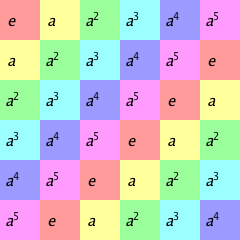`The inverse and basis matrices are_|_I_A_B_C_D_EI| I A B C D EE| E I A B C D     AE = ID| D E I A B C     BD = IC| C D E I A B     C^2 = IB| B C D E I AA| A B C D E I    1 0 0 0 0 0        0 1 0 0 0 0        0 0 1 0 0 0    0 1 0 0 0 0        0 0 1 0 0 0        0 0 0 1 0 0I = 0 0 1 0 0 0    A = 0 0 0 1 0 0    B = 0 0 0 0 1 0    0 0 0 1 0 0        0 0 0 0 1 0        0 0 0 0 0 1    0 0 0 0 1 0        0 0 0 0 0 1        1 0 0 0 0 0    0 0 0 0 0 1        1 0 0 0 0 0        0 1 0 0 0 0        0 0 0 1 0 0        0 0 0 0 1 0        0 0 0 0 0 1    0 0 0 0 1 0        0 0 0 0 0 1        1 0 0 0 0 0C = 0 0 0 0 0 1    D = 1 0 0 0 0 0    E = 0 1 0 0 0 0    1 0 0 0 0 0        0 1 0 0 0 0        0 0 1 0 0 0    0 1 0 0 0 0        0 0 1 0 0 0        0 0 0 1 0 0    0 0 1 0 0 0        0 0 0 1 0 0        0 0 0 0 1 0`

#### S3 - Symmetric Group (Permutation Group) With Six Elements

S3 is the other group with six elements. S3 (3! = 6) represents the set of all possible permutations of three elements.
The default table from GAP is
`gap> StructureDescription(SmallGroup(6,1));"S3"gap> MultiplicationTable(SmallGroup(6,1));                              _|_I_A_B_C_D_E[ [ 1, 2, 3, 4, 5, 6 ],       I| I A B C D E   [ 2, 1, 4, 3, 6, 5 ],       A| A I C B E D  [ 3, 6, 5, 2, 1, 4 ],       B| B E D A I C  [ 4, 5, 6, 1, 2, 3 ],       C| C D E I A B  [ 5, 4, 1, 6, 3, 2 ],       D| D C I E B A  [ 6, 3, 2, 5, 4, 1 ] ]      E| E B A D C I`
From Group Explorer, we have the generator set  <a,b:a3=b2=1,bab=a-1>
In the multiplication table from Group Explorer, we see letters r for rotate and f for flip.
These tables below have been organized by subgroup, with cosets.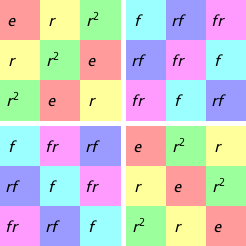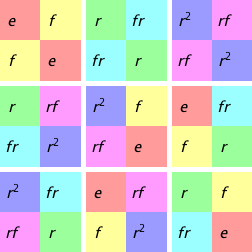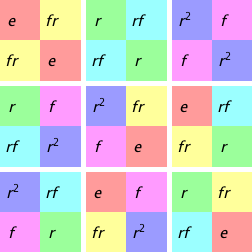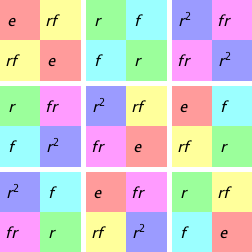Inverse elements and powers
`_|_I_A_B_C_D_EI| I A B C D E     A^2 = I              1/A = IA| A I C B E D     B^2 = D    B^3 = I   1/B = DD| D C I E B A     C^2 = I              1/C = CC| C D E I A B     D^2 = B    D^3 = I   1/D = BB| B E D A I C     E^2 = I              1/E = EE| E B A D C I`
Matrix Representations
`    1 0 0 0 0 0        0 1 0 0 0 0        0 0 1 0 0 0    0 1 0 0 0 0        1 0 0 0 0 0        0 0 0 1 0 0I = 0 0 1 0 0 0    A = 0 0 0 0 0 1    B = 0 0 0 0 1 0    0 0 0 1 0 0        0 0 0 0 1 0        0 0 0 0 0 1    0 0 0 0 1 0        0 0 0 1 0 0        1 0 0 0 0 0    0 0 0 0 0 1        0 0 1 0 0 0        0 1 0 0 0 0        0 0 0 1 0 0        0 0 0 0 1 0        0 0 0 0 0 1    0 0 1 0 0 0        0 0 0 0 0 1        0 0 0 0 1 0C = 0 1 0 0 0 0    D = 1 0 0 0 0 0    E = 0 0 0 1 0 0    1 0 0 0 0 0        0 1 0 0 0 0        0 0 1 0 0 0    0 0 0 0 0 1        0 0 1 0 0 0        0 1 0 0 0 0    0 0 0 0 1 0        0 0 0 1 0 0        1 0 0 0 0 0`